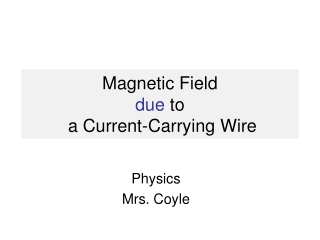DownloadDownload PresentationMagnetic Field due to a Current-Carrying Wire

# Magnetic Field due to a Current-Carrying Wire

Download Presentation## Magnetic Field due to a Current-Carrying Wire

- - - - - - - - - - - - - - - - - - - - - - - - - - - E N D - - - - - - - - - - - - - - - - - - - - - - - - - - -
##### Presentation Transcript

1. Magnetic Field due to a Current-Carrying Wire Physics Mrs. Coyle

2. A Current-Carrying Wire Causes a Magnetic Field Around it.

3. Hans Christian Oersted, 1820

4. Right Hand Curl Rule

5. Magnetic Fields of Long Current-Carrying Wires B = mo I 2p r I = current through the wire (Amps) r = distance from the wire (m) mo = permeability of free space = 4p x 10-7 T m / A B = magnetic field strength (Tesla) I

6. Example 1 • What is the magnitude of the magnetic field created by a 0.25A current at a distance of 50cm from the wire? • Answer: 1x10-7 Tesla

7. Magnetic Field of a Current Carrying Wire • http://www.walter-fendt.de/ph14e/mfwire.htm

8. Magnetic Field in the Center of a Current-Carrying Loop (Ring) B = mo I 2r

9. Note • The overall shape of the magnetic field of the circular loop is similar to the magnetic field of a bar magnet.

10. Magnetic Field at Center of a SolenoidB = mo NIL N: Number of turns L: Length n=N/L ------------------------L----------------

11. Force of Attraction Between Two Long Current-Carrying Wires F= mo I1 I2 L 2p d d : distance between wires I : current L: length of wire

12. Note • If the wires have currents in opposite directions, the wires repel each other.

13. Example 2 • What is the force of attraction felt by two 4m long current-carrying wires carrying currents of 2A and 3A, that are 60cm from each other? • Answer: 8x10-6 N